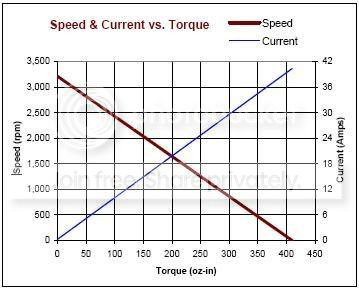Author Topic: speed and current vs torque  (Read 17373 times)

0 Members and 1 Guest are viewing this topic.vidamspeed and current vs torque
« on: June 04, 2008, 12:36:37 PM »
don't believe I understand the graph for a DC motor.When there is no external load (i.e. torque) applied to the motor then the motor runs at maximum speed, zero current, and I assume max voltage. I don't understand how that is even possible since P=I*V. maybe it approximates a small current and are calling it neglible.

confused...

-Melaniebens

• Expert Roboticist
• Supreme Robot
•• Posts: 335Re: speed and current vs torque
« Reply #1 on: June 04, 2008, 12:53:53 PM »
The power of an ideal motor that experiences no torque is zero.  The reason this is theoretically possible in an idea motor is that a motor spins when a voltage is applied to it and a motor generates a voltage when it spins.  If you have no power losses, the voltage generated by the motor as it spins exactly cancels the voltage that is spinning the motor, and you have no current passing through the coils.  Since it is never possible to have a lossless system (there will always be friction and the coils will always have some resistance), you won't ever see this in a real-world motor.

- BenvidamRe: speed and current vs torque
« Reply #2 on: June 04, 2008, 12:59:05 PM »
Is there such a thing as a Speed and voltage vs torque curve?benjiRe: speed and current vs torque
« Reply #3 on: June 04, 2008, 02:40:46 PM »

the laplace force increases when current increases (according to laplace force theory)
dF=I .* (VECTOR (Dl)  ^  VECTOR(B))
I is current
dl minimum length of wire
B magnetic field

you have also

power = force * velocity

V.*I = INTEGRAL OF (dF) * velocity

guess u can calculate velocity nowthis also might be usefull

http://www.ajdesigner.com/phppower/power_equation_velocity_force.php
« Last Edit: June 04, 2008, 02:42:26 PM by benji »
good ol' BeNNyvidamRe: speed and current vs torque
« Reply #4 on: July 14, 2008, 09:29:21 AM »
Here is a simple linear equation that relates Power to torque and rotational speed. You don't have to do integral math of differential variables.

Power (kW) = ( torque (Nm) times 2pi times rotational speed (rpm) ) / 60000

Substituing Power = V * I

You can then solve for I (current) and you get how current varies with respect to torque, voltage, and rotational speed.

http://en.wikipedia.org/wiki/TorqueAdminRe: speed and current vs torque
« Reply #5 on: July 14, 2008, 09:52:58 AM »
please no more double postinghttp://www.societyofrobots.com/robotforum/index.php?topic=4653.0vidamRe: speed and current vs torque
« Reply #6 on: July 28, 2008, 11:46:22 PM »
I have 2 words for this post:
STALL TORQUE

This cleared up everything for me. I no longer have questions for this post.

http://en.wikipedia.org/wiki/Stall_torque
http://www.faulhaber-group.com/n390432/n.html